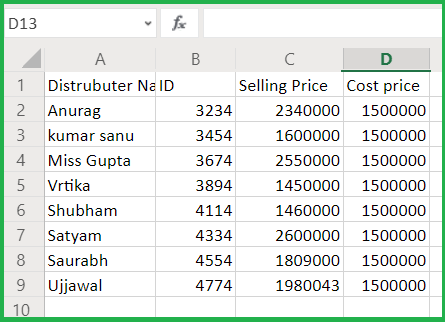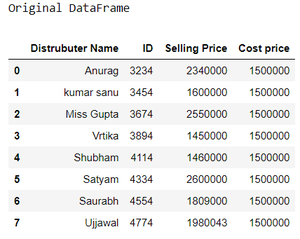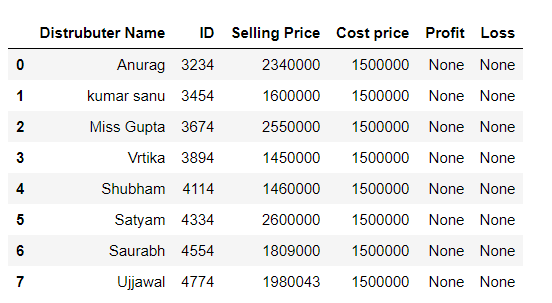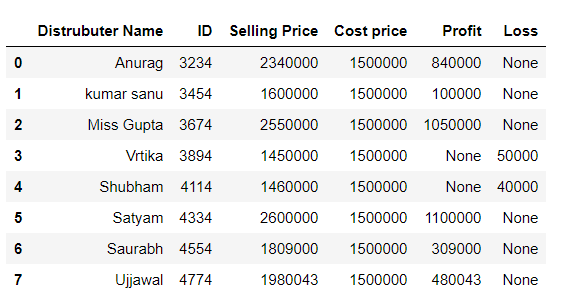# Find the profit and loss in the given Excel sheet using Pandas

In these articles, we will discuss how to extract data from the Excel file and find the profit and loss at the given data. Suppose our Excel file looks like then we have to extract the Selling Price and Cost Price from the column and find the profit and loss and store it into a new DataFrame column.So, Let’s discuss the approach:

Step 1: Import the required module and read data from excel.

## Python3

 `# importing module ` ` `  `import` `pandas as pd; ` ` `  `# Creating df ` `# Reading data from Excel ` `data ``=` `pd.read_excel(``"excel_work/book_sample.xlsx"``); ` ` `  `print``(``"Original DataFrame"``) ` `data`

Output :Step 2: Create a new column in DataFrame for store Profit and Loss

## Python3

 `# Create column for profit and loss ` `data[``'Profit'``]``=` `None` `data[``'Loss'``]``=` `None` ` `  `data `

Output :Step 3: Set Index for Selling price, Cost price, Profit, and loss for accessing the DataFrame columns

## Python3

 `# set index ` `index_selling ``=` `data.columns.get_loc(``'Selling Price'``) ` `index_cost ``=` `data.columns.get_loc(``'Cost price'``) ` `index_profit ``=` `data.columns.get_loc(``'Profit'``) ` `index_loss ``=` `data.columns.get_loc(``'Loss'``) ` ` `  `print``(index_selling, index_cost, index_profit, index_loss) `

Output :

```2 3 4 5
```

Step 4: Compute profit and loss according to there each column index.

```Profit = Selling price - Cost price
Loss = Cost price - Selling price```

## Python3

 `# Loop for accessing every index in DataFrame ` `# and compute Profit and loss ` `# and store into new column in DataFrame ` `for` `row ``in` `range``(``0``, ``len``(data)): ` `    ``if` `data.iat[row, index_selling] > data.iat[row, index_cost]: ` `        ``data.iat[row, index_profit] ``=` `data.iat[row, ` `                                               ``index_selling] ``-` `data.iat[row, index_cost] ` `    ``else``: ` `        ``data.iat[row, index_loss] ``=` `data.iat[row, ` `                                             ``index_cost]``-``data.iat[row, index_selling] ` `data `

Output :Whether you're preparing for your first job interview or aiming to upskill in this ever-evolving tech landscape, GeeksforGeeks Courses are your key to success. We provide top-quality content at affordable prices, all geared towards accelerating your growth in a time-bound manner. Join the millions we've already empowered, and we're here to do the same for you. Don't miss out - check it out now!

Previous
Next